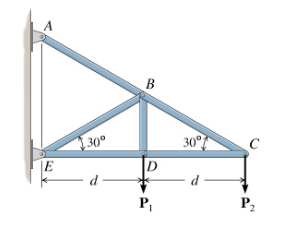# Problem: Consider the truss shown in the diagram. The applied forces are P =570 lb and P2 = 430 lb and the distance is d=8.50 ft. A. For this truss, what joint is the best place to start the analysis?B. Find all forces in all joints C, D, B C. What are the forces in the two members CB and CD? D. Having found FCD in the analysis of joint C, there are now only two unknown forces acting on joint. Determine the two unknown forces on joint D. P, P, 3

###### FREE Expert Solution
91% (240 ratings)###### Problem Details

Consider the truss shown in the diagram. The applied forces are P =570 lb and P2 = 430 lb and the distance is d=8.50 ft.A. For this truss, what joint is the best place to start the analysis?

B. Find all forces in all joints C, D, B

C. What are the forces in the two members CB and CD?

D. Having found FCD in the analysis of joint C, there are now only two unknown forces acting on joint. Determine the two unknown forces on joint D. P, P, 3